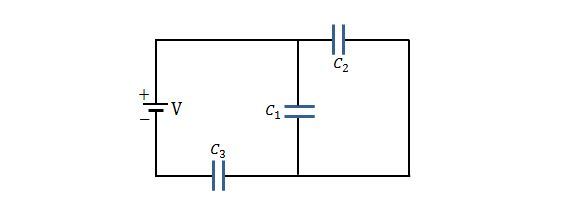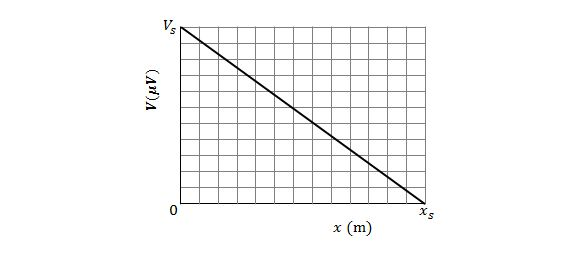Electricity and Magnetism

# Problem solving - Circuit elementsIf a potential difference of $V=115\text{ V}$ is applied across a capacitor arrangement with capacitances $C_1=10\,\mu\text{F},$ $C_2=6\,\mu\text{F}$ and $C_3=15\,\mu\text{F},$ as shown in the above figure, what is the approximate stored energy $U_3$ for capacitor 3?

A $12.0\text{ V}$ battery is connected to a series of $n$ capacitors, each of capacitance $2\,\mu\text{F}.$ If the total stored energy is $36.0\,\mu\text{J},$ what is $n?$

Approximately how much electrical energy is transferred to thermal energy in 3 hours by an electrical resistance of $350.0 \,\Omega$ when the potential applied across it is $90.0\text{ V}?$

Consider a circuit where a potential difference of $0.80 \text{ mV}$ is set up across a resistor with length $2.50 \text{ cm}$ and radius $1.30 \text{ mm}.$ Approximately how much charge drifts through the cross section of the resistor in $30.00 \text{ s},$ assuming that the resistivity of the resistor is $2.35 \times 10^{-8} \,\Omega\cdot\text{m}?$The above figure shows the electric potential $V(x)$ along a copper wire as a function of position $x.$ The radius of the copper wire is $3.0\text{ mm}.$ If $V_s=11.0\,\mu\text{V}$ and $x_s=3.00\text{ m}$ in the figure, what is the approximate current in the wire?

The resistivity of copper is $1.69 \times 10^{-8}\,\Omega\cdot\text{m}.$

×